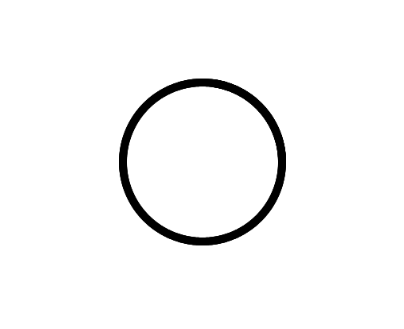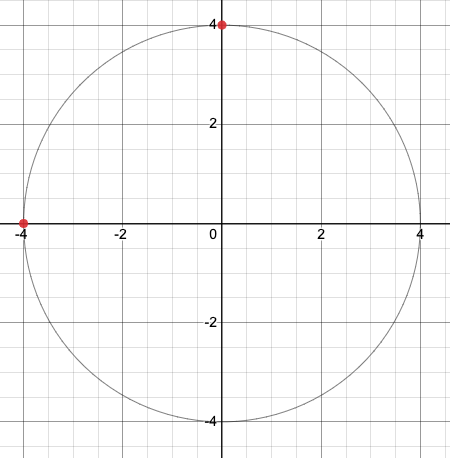# How to make a full-fledged camera

hello! introduction stuff CAMERAS ARE COOL
they work well with all sorts of 2d games , and people already know how to implement a basic camera
you only need two global variables, two object variables, and an understanding of subtraction

see? I just made an example with like four blocks

### thing is

that’s only positioning
sure, zooming is not harder to implement, just multiply values and scale stuff but WHAT ABOUT ROTATION?
this is where things get tricky.

real quick have a fun poll

Do you know how to program a camera?
• With positioning
• With zooming
• With rotating
• None
0 voters

this poll is anonymous by the way

# just make a circle

circles are easy to make, you take your basic cos() and sin() functions with an extra variable to determine where we are drawing

and when we run we get

…okay don’t forget to offset it that is a good steptrail art aside, we already know how to draw a basic circle 
only thing, how do we implement it with positions??

# offsetting

if we look at a line rotating, and mark points, we can see that bigger and bigger circles can resolve this

the same can apply for the y axis too
buuut now we have a problem

we can easily determine a radius for any point ON either axisbut things get tricky if it’s NOT
sure, we can use good ol’ pythagoream theorem to get the radius, but what about knowing WHERE to place it? 


# circles and circles

what if I told you
we use cos() and sin()
TWICE
wow so revolutionary

what’s nice about this method is that both circles rotate, accounting for the X and Y position of the dot 

what is this math wise though?

(cos(r) * 2 + cos(r + 90) * 4, sin(r) * 2 + sin(r + 90) * 4)
ok lets break this down

we’re defining a point here,
cos(r) and sin(r) account for the first circle, while * 2 is the dot’s X position
+ cos(r + 90) and + sin(r + 90) account for the second circle, rotating by 90 degrees so it points upwards (also * 4 for the Y position of the dot)
adding both together, we get the final position

now we have:
(r is camera rotation, x and cx are the object X and camera X positions, y and cy are the object Y and camera Y positions)

(cos(r) * (x - cx) + cos(r + 90) * (y - cy),
sin(r) * (x - cx) + sin(r + 90) * (y - cy))

# zooming!!

obviously, rotations is enough for most games, but zooming is also great as well
it’s not hard to implement actually, just multiply the x - cx and y - cy part by the camera’s zoom

(z is the camera’s zoom)
(cos(r) * ((x - cx) * z) + cos(r + 90) * (y - cy),
sin(r) * ((x - cx * z)) + sin(r + 90) * (y - cy))

everything here was taught to me by myself! if there’s any corrections you would suggest or need further explanation on something please do tell me!
regular feedback is welcome too!

# oh yeah by the way do remember to offset everything by half the width and height of the screen

1. 3d as well but this is hopscotch ↩︎

2. or now know ↩︎

3. as in like, after we rotate the camera where does it go ↩︎

4. there’s probably a math function exactly for this but I’m not the ↩︎

5. if that makes sense? ↩︎

9 Likes

ive done zoom + rotate follow cam in the past and it was pain

super awesome tutorial!!

7 Likes

thanks! funny you say it was pain though since this wasn’t that hard to figure out and then conceptualize lol

7 Likes

Having learned trigonometry, it’s really easy as a concept, but since I haven’t done it before I don’t know how hard it would be to code and debug (mostly debug) in Hopscotch. Really cool tutorial though!

9 Likes

is there any project for this?

I honestly don’t really know what it’s talking about so well

9 Likes
6 Likes

rip it’s currently stuck in the filter :((

5 Likes

aaaahhh

5 Likes

Looks like it was caught by the word Zoom (like video calls) so not sure if that’d come out on its own. Might want to put that in the filter topic then

6 Likes

@Pandy-y project got unfiltered

4 Likes

So basically 3d?

4 Likes

what?

2 Likes

What if I want only a partially-fledged camera?

3 Likes

…just zooming I assume? I’m not sure what you mean by this but that’s done by multiplying the x - cx and y - cy by the camera’s zoom

2 Likes

I don’t know I just am too lazy to read

2 Likes

in that case, defledge it to your preference before proceeding

4 Likes